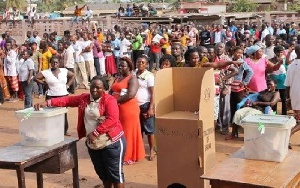You are here: HomeWallOpinionsArticles2020 12 05Article 1127174

Opinions of Saturday, 5 December 2020

Columnist: Michael Graham

# Numerological analysis for 2020 electionsFile photo: A voter casting her vote

Numerology is the science of numbers which can reveal individual energy and vibration.

The birth date reveals a lot and cannot be underestimated. The birthdate for John Dramani Mahama is 29/11/1958.

To calculate his destiny number, the birthdate is reduced to a single digit, thus 2+9+1+1+1+9+5+8=36 =3+6=9
His life path number is 9
Now let us see what happened in 2012.

To calculate his personal year number, we replace the year date in the birthdate to 2012 and reduce it to a single digit.

Hence 29/11/2012.
Reducing it to a single digit,
2+9+1+1+2+0+1+2=18=1+8=9

The personal year number harmonised with the destiny number and he won the elections.
Let’s see 2016

The birth date of President Nana Akufo Addo is 29/03/1944.
His destiny number again calculated as
2+9+3+1+9+4+4=32=3+2=5

His destiny number is 5.
His personal year number for 2016
29/3/2016
2+9+3+2+0+1+6=23=2+3=5

The personal year number synchronized with destiny number and he won.
In 2020, the scenario looks a bit different.
Let us calculate their personal year vibrations.
John Mahama,
29/11/2020
2+9+1+1+2+0+2+0=17=1+7=8
29/3/2020
2+9+3+2+0+2+0=18=9
Their personal year numbers are different from their destiny numbers.

Personal year 8 for John Mahama as against 9 for President Nana Akufo Addo.
An amazing scenario indeed.
The number 8 and 9 are both numbers of leadership, authority and power.

A contentious energy is generated when these two vibrations meet.
A real fight for supremacy or power.

Vibration 9 is a pure power vibration and indicates the completion of a cycle and the ushering or birth of a new cycle.

The personal year number 9 enters a new vibration of 1 from the first of September in the year.
President Nana Akufo Addo assumed the personal year number 9 in March per his birthdate.

His vibration after 1st September is vibration 1.
He swings in both vibration 9 and 1.

This gives him exclusive advantage over John Mahama whose personal year number for the year is 8, which effect started on his birthdate 29th November.

We can also calculate the energy available for the day of elections to ascertain who is more harmonious with the day's energy.
7/12/2020
7+1+2+2+0+2+0=14=5

The energy for the day is 5 and his is in harmony with the destiny number 5 for the President.

Nana Akufo has a high chance of winning the 2020 Presidential elections.

### Entertainment